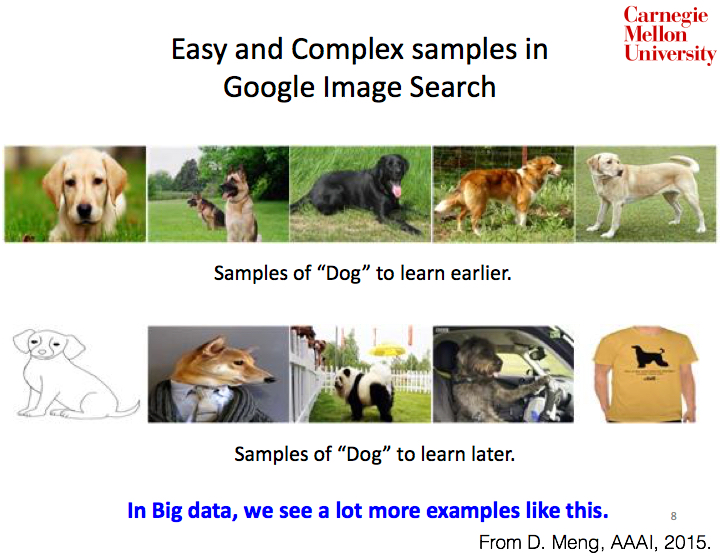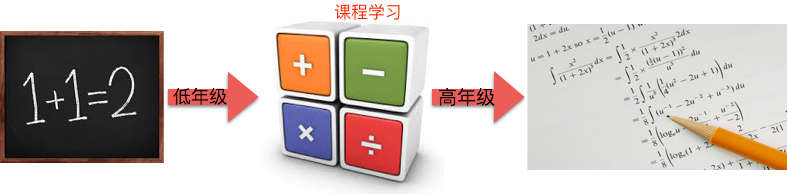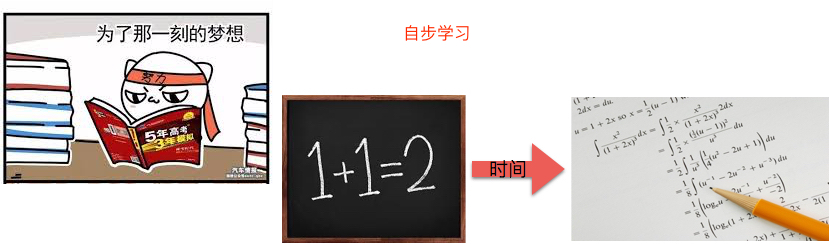# 机器学习-自步学习

Posted by Longfei Han on July 24, 2017

## 1. 自步学习## 3. SPL

$w_{t+1} = argmin(r(w) + \sum_{i=1}^n f(x_i, y_i, w)).$

$(w_{t+1}, v_{t+1}) = argmin(r(w) + \sum_{i=1}^n v_i f(x_i, y_i, w) - \frac{1}{K} \sum_{i=1}^n v_i).$

## 5. 参考文献

 Deyu Meng老师主页.

 Q. Zhao, D. Meng, L. Jiang, Q. Xie, Z. Xu, and A.G. Hauptmann. Self-Paced Learning for Matrix Factorization. AAAI, 2015, 3196–3202.

 L. Jiang, D. Meng, T. Mitamura, and A.G. Hauptmann. Easy samples first: Self-paced reranking for zero-example multimedia search. MM, 2014, 547–556.

 D. Zhang, D. Meng, and J. Han. Co-saliency detection via a self-paced multiple-instance learning framework. IEEE TPAMI, 2016, online published.

 L. Jiang, D. Meng, S.I. Yu, Z. Lan, S. Shan, and A.G. Hauptmann. Self-paced learning with diversity. NIPS, 2014, 2078–2086.

 J.S. Supancic and D. Ramanan. Self-paced learning for long-term tracking. CVPR, 2013, 2379–2386.

 L. Han, D. Zhang, D. Huang, X. Chang, J. Ren. IJCAI, 2017, accepted.

 Lu Jiang SPLD主页.

 Dingwen Zhang主页.

 Kumar M P, Packer B, Koller D. Self-paced learning for latent variable models. NIPS, 2010, 1189-1197.